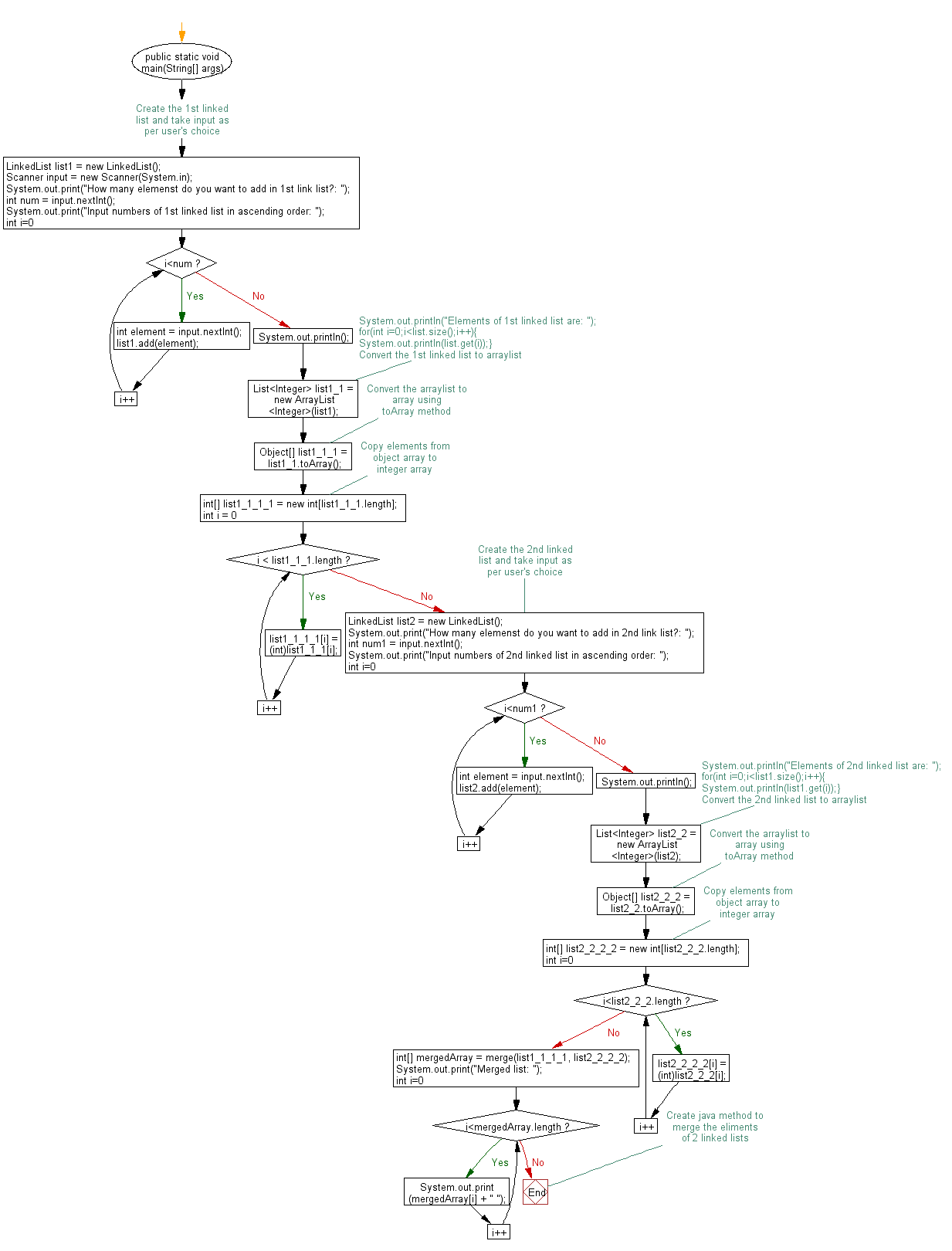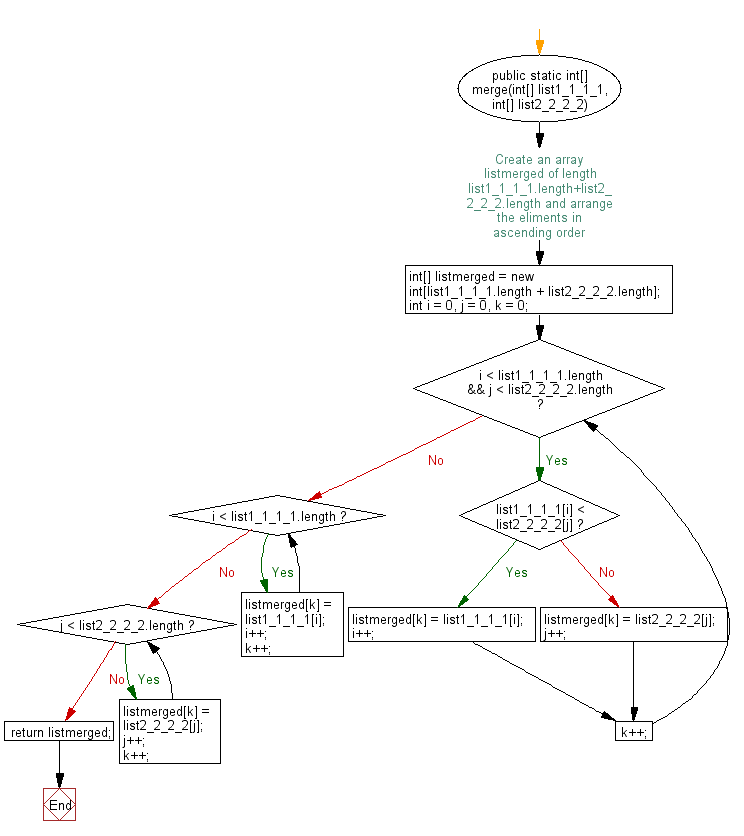# Java: Merge two sorted (ascending) linked lists in ascending order

## Java Basic: Exercise-207 with Solution

Write a Java program to merge two sorted (ascending) linked lists in ascending order.

Sample Solution:

Java Code:

``````// Importing the java.util package to use LinkedList, Scanner, ArrayList, and List
import java.util.*;

// Defining the Main class
class Main {
// Main method, the entry point of the program
public static void main(String[] args) {
// Creating the 1st linked list

// Creating a Scanner object for user input
Scanner input = new Scanner(System.in);

// Prompting the user to input the number of elements for the 1st linked list
System.out.print("How many elements do you want to add in the 1st linked list?: ");

// Reading the number of elements from the user
int num = input.nextInt();

// Prompting the user to input numbers for the 1st linked list in ascending order
System.out.print("Input numbers of the 1st linked list in ascending order: ");

for (int i = 0; i < num; i++) {
int element = input.nextInt();
}
System.out.println();

// Converting the 1st linked list to ArrayList
List<Integer> list1_1 = new ArrayList<Integer>(list1);
Object[] list1_1_1 = list1_1.toArray();
int[] list1_1_1_1 = new int[list1_1_1.length];
for (int i = 0; i < list1_1_1.length; i++)
list1_1_1_1[i] = (int) list1_1_1[i];

// Creating the 2nd linked list

// Prompting the user to input the number of elements for the 2nd linked list
System.out.print("How many elements do you want to add in the 2nd linked list?: ");

// Reading the number of elements from the user
int num1 = input.nextInt();

// Prompting the user to input numbers for the 2nd linked list in ascending order
System.out.print("Input numbers of the 2nd linked list in ascending order: ");

for (int i = 0; i < num1; i++) {
int element = input.nextInt();
}
System.out.println();

// Converting the 2nd linked list to ArrayList
List<Integer> list2_2 = new ArrayList<Integer>(list2);
Object[] list2_2_2 = list2_2.toArray();
int[] list2_2_2_2 = new int[list2_2_2.length];
for (int i = 0; i < list2_2_2.length; i++)
list2_2_2_2[i] = (int) list2_2_2[i];

// Merging the two lists using the merge method
int[] mergedArray = merge(list1_1_1_1, list2_2_2_2);

// Printing the merged list
System.out.print("Merged list: ");
for (int i = 0; i < mergedArray.length; i++) {
System.out.print(mergedArray[i] + " ");
}
}

// Method to merge two sorted arrays
public static int[] merge(int[] list1_1_1_1, int[] list2_2_2_2) {
// Creating an array to store the merged list
int[] listMerged = new int[list1_1_1_1.length + list2_2_2_2.length];

// Initializing indices for both lists and the merged list
int i = 0, j = 0, k = 0;

// Merging the two lists
while (i < list1_1_1_1.length && j < list2_2_2_2.length) {
if (list1_1_1_1[i] < list2_2_2_2[j]) {
listMerged[k] = list1_1_1_1[i];
i++;
} else {
listMerged[k] = list2_2_2_2[j];
j++;
}
k++;
}

// Copying the remaining elements of list1_1_1_1, if any
while (i < list1_1_1_1.length) {
listMerged[k] = list1_1_1_1[i];
i++;
k++;
}

// Copying the remaining elements of list2_2_2_2, if any
while (j < list2_2_2_2.length) {
listMerged[k] = list2_2_2_2[j];
j++;
k++;
}

// Returning the merged list
return listMerged;
}
}
```
```

Output:

```How many elements do you want to add in 1st linked list?:  3
Input numbers of 1st linked list in ascending order:  1 2 3

How many elements do you want to add in 2nd linked list?:  3
Input numbers of 2nd linked list in ascending order:  3 4 5

Merged list: 1 2 3 3 4 5
```

Flowchart:Java Code Editor: